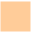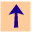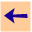6.4 Why? Questions

## 6.4 Why? Questions

If you find yourself at a particular subgoal, it is often useful to find out why that subgoal is being proved.

When you ask why an atom was being asked, CILOG produces the instance of the rule in the knowledge base with the atom in the body, such that the head was trying to be proved. This is of the form:

```h <-
1: a_1
2: a_2
...
** j: a_j
...
k: a_k
```
This means that aj is the atom that is being asked, the atoms a1...aj-1 have been proved, and aj+1...ak have still to be proved. When this is presented you can ask one of:
how i.
where i is an integer 1 <= i < j. This means that you want to see how ai was proved. This enters the how dialog.
how j.
This means that you want to see how CILOG is trying to prove aj (the atom you have previously asked why about). This returns to the rule with aj in the body.
why.
to see the rule with h in the body.
prompt.
help.
for a list of the available commands.
Note that you can't ask how i for i>j as there is no proof tree for the atoms aj+1...ak. Also, CILOG does not guarantee that the rule given will have the atoms in the body in the same order as in the knowledge base; CILOG is free to change the order of atoms in a body as long as this doesn't introduce an error.

Example. The following shows a trace of a looping program that reaches the depth bound. We first increase the depth-bound, and then explore where the depth-bound was reached.

```cilog: tell a <- b & c & d.
cilog: tell b <- e.
cilog: tell e.
cilog: tell c <- f & d & g.
cilog: tell f <- b & h.
cilog: tell h.
cilog: tell d <- a.
Query failed due to depth-bound 30.
[New-depth-bound,where,ok,help]: 50.
Query failed due to depth-bound 50.
[New-depth-bound,where,ok,help]: where.
Depth-bound reached. Current subgoal: e
[fail,succeed,proceed,why,ok,help]: why.
e is used in the rule
b <-
** 1: e
[Number,why,help,ok]: why.
b is used in the rule
a <-
** 1: b
2: c
3: d
[Number,why,help,ok]: why.
a is used in the rule
d <-
** 1: a
[Number,why,help,ok]: why.
d is used in the rule
c <-
1: f
** 2: d
3: g
[Number,why,help,ok]: how 1.
f <-
1: b
2: h
How? [Number,up,retry,ok,help]: how 2.
h is a fact
f <-
1: b
2: h
How? [Number,up,retry,ok,help]: up.
d is used in the rule
c <-
1: f
** 2: d
3: g
[Number,why,help,ok]: why.
c is used in the rule
a <-
1: b
** 2: c
3: d
[Number,why,help,ok]: ok.
Depth-bound reached. Current subgoal:e
[fail,succeed,proceed,why,ok,help]: fail.6.4 Why? Questions